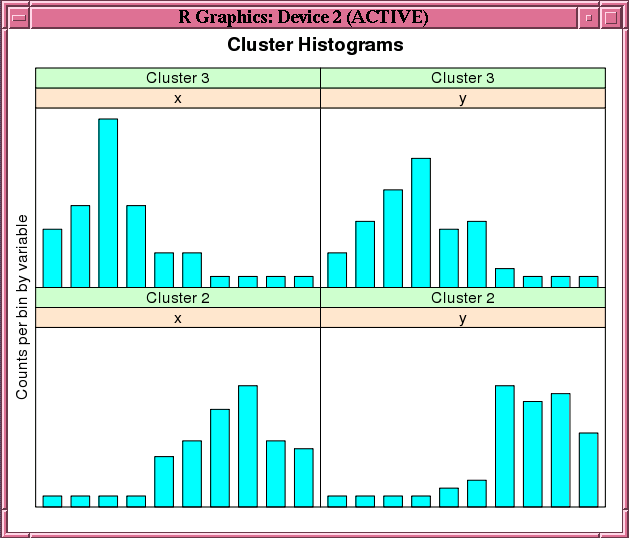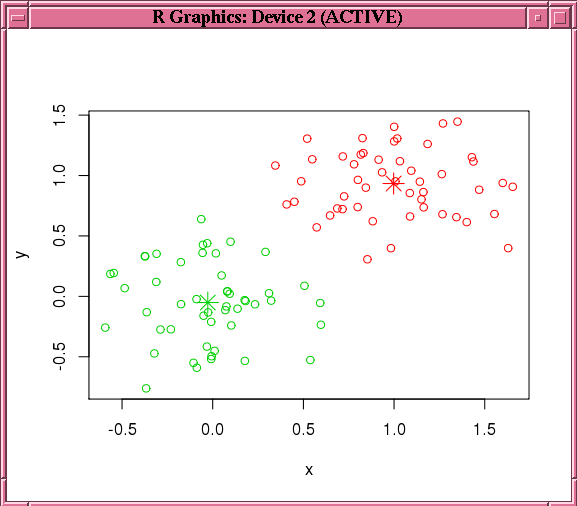# 4.2.6 Building a k-Means Model

The `ore.odmKM` function uses the Oracle Data Mining k-Means (KM) algorithm, a distance-based clustering algorithm that partitions data into a specified number of clusters. The algorithm has the following features:

• Several distance functions: Euclidean, Cosine, and Fast Cosine distance functions. The default is Euclidean.

• For each cluster, the algorithm returns the centroid, a histogram for each attribute, and a rule describing the hyperbox that encloses the majority of the data assigned to the cluster. The centroid reports the mode for categorical attributes and the mean and variance for numeric attributes.

For information on the `ore.odmKM` function arguments, invoke `help(ore.odmKM)`.

Example 4-15 Using the ore.odmKM Function

This example demonstrates the use of the `ore.odmKMeans` function. The example creates two matrices that have 100 rows and two columns. The values in the rows are random variates. It binds the matrices into the `matrix` `x`, then coerces `x` to a `data.frame` and pushes it to the database as `x_of`, an `ore.frame` object. The example next invokes the `ore.odmKMeans` function to build the KM model, `km.mod1`. It then invokes the `summary` and `histogram` functions on the model. Figure 4-2 shows the graphic displayed by the `histogram` function.

Finally, the example makes a prediction using the model, pulls the result to local memory, and plots the results.Figure 4-3 shows the graphic displayed by the `points` function.

```x <- rbind(matrix(rnorm(100, sd = 0.3), ncol = 2),
matrix(rnorm(100, mean = 1, sd = 0.3), ncol = 2))
colnames(x) <- c("x", "y")
x_of <- ore.push (data.frame(x))
km.mod1 <- NULL
km.mod1 <- ore.odmKMeans(~., x_of, num.centers=2)
summary(km.mod1)
histogram(km.mod1)
# Make a prediction.
km.res1 <- predict(km.mod1, x_of, type="class", supplemental.cols=c("x","y"))
# Pull the results to the local memory and plot them.
km.res1.local <- ore.pull(km.res1)
plot(data.frame(x=km.res1.local\$x, y=km.res1.local\$y),
col=km.res1.local\$CLUSTER_ID)
points(km.mod1\$centers2, col = rownames(km.mod1\$centers2), pch = 8, cex=2)
supplemental.cols=c("x","y")), 3)
```
Listing for Example 4-15
```R> x <- rbind(matrix(rnorm(100, sd = 0.3), ncol = 2),
+             matrix(rnorm(100, mean = 1, sd = 0.3), ncol = 2))
R> colnames(x) <- c("x", "y")
R> x_of <- ore.push (data.frame(x))
R> km.mod1 <- NULL
R> km.mod1 <- ore.odmKMeans(~., x_of, num.centers=2)
R> summary(km.mod1)

Call:
ore.odmKMeans(formula = ~., data = x_of, num.centers = 2)

Settings:
value
clus.num.clusters            2
block.growth                 2
conv.tolerance            0.01
distance             euclidean
iterations                   3
min.pct.attr.support       0.1
num.bins                    10
split.criterion       variance
prep.auto                   on

Centers:
x           y
2  0.99772307  0.93368684
3 -0.02721078 -0.05099784
R> histogram(km.mod1)
R> # Make a prediction.
R> km.res1 <- predict(km.mod1, x_of, type="class", supplemental.cols=c("x","y"))
x          y CLUSTER_ID
1 -0.03038444  0.4395409          3
2  0.17724606 -0.5342975          3
3 -0.17565761  0.2832132          3
# Pull the results to the local memory and plot them.
R> km.res1.local <- ore.pull(km.res1)
R> plot(data.frame(x=km.res1.local\$x, y=km.res1.local\$y),
+                  col=km.res1.local\$CLUSTER_ID)
R> points(km.mod1\$centers2, col = rownames(km.mod1\$centers2), pch = 8, cex=2)
supplemental.cols=c("x","y")), 3)
'2'       '3'           x          y CLUSTER_ID
1 8.610341e-03 0.9913897 -0.03038444  0.4395409          3
2 8.017890e-06 0.9999920  0.17724606 -0.5342975          3
3 5.494263e-04 0.9994506 -0.17565761  0.2832132          3
```

Figure 4-2 shows the graphic displayed by the invocation of the `histogram` function in Example 4-15.

Figure 4-2 Cluster Histograms for the km.mod1 ModelDescription of "Figure 4-2 Cluster Histograms for the km.mod1 Model"

Figure 4-3 shows the graphic displayed by the invocation of the `points` function in Example 4-15.

Figure 4-3 Results of the points Function for the km.mod1 ModelDescription of "Figure 4-3 Results of the points Function for the km.mod1 Model"